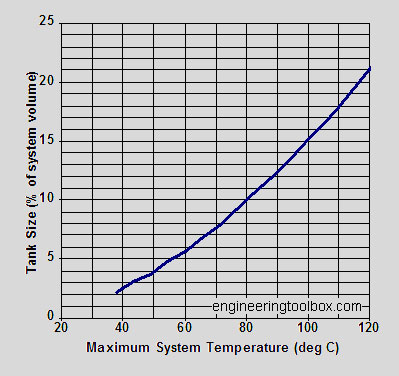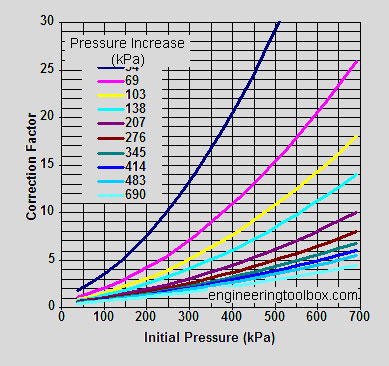# Closed Expansion Tanks

In a closed expansion tank the pressure in the system is maintained partly by compressed air. The expansion tank volume can be expressed as:

Vt = Ve pw / (pw – pi)

where

Vt = volume of expansion tank (m3)
Ve = volume by which water contents expands (m3)
pw = absolute pressure of tank at working temperature – operating system (kN/m2)
pi = absolute pressure of cold tank at filling – non operating system (kN/m2)
The expanding volume may be expressed as:
Ve = Vw (?i – ?w) / ?w
where
Vw = volume of water in the system (m3)
?i = density of cold water at filling temperature (kg/m3)
?w = density of water at working temperature (kg/m3)
The working pressure of the system – pw – should be so that the working pressure at highest point of the system corresponds to the boiling point 10°C above the working temperature.
pw = working pressure at highest point

• + static pressure difference between highest point and tank
• +/- pump pressure (+/- according the position of the pump)

The diagram below can be used to estimate the size of a closed expansion tank at low temperatures.

• initial temperature 10°C
• initial pressure 70 kPa
• maximum operating pressure 200 kPaFor initial and maximum pressures different from above – multiply the tank size with the a correction factor estimated from the diagram below:Ps> Open expansion tanks should be prefered in Solid Fuel fired systems. In the event of a blackout, circulation pump and control systems are inoperable and the fuel in chamber continous to burn. This causes heating water to reach undesired temperatures and might damage the closed expansion tanks. (Please see Standard EN 303-5).